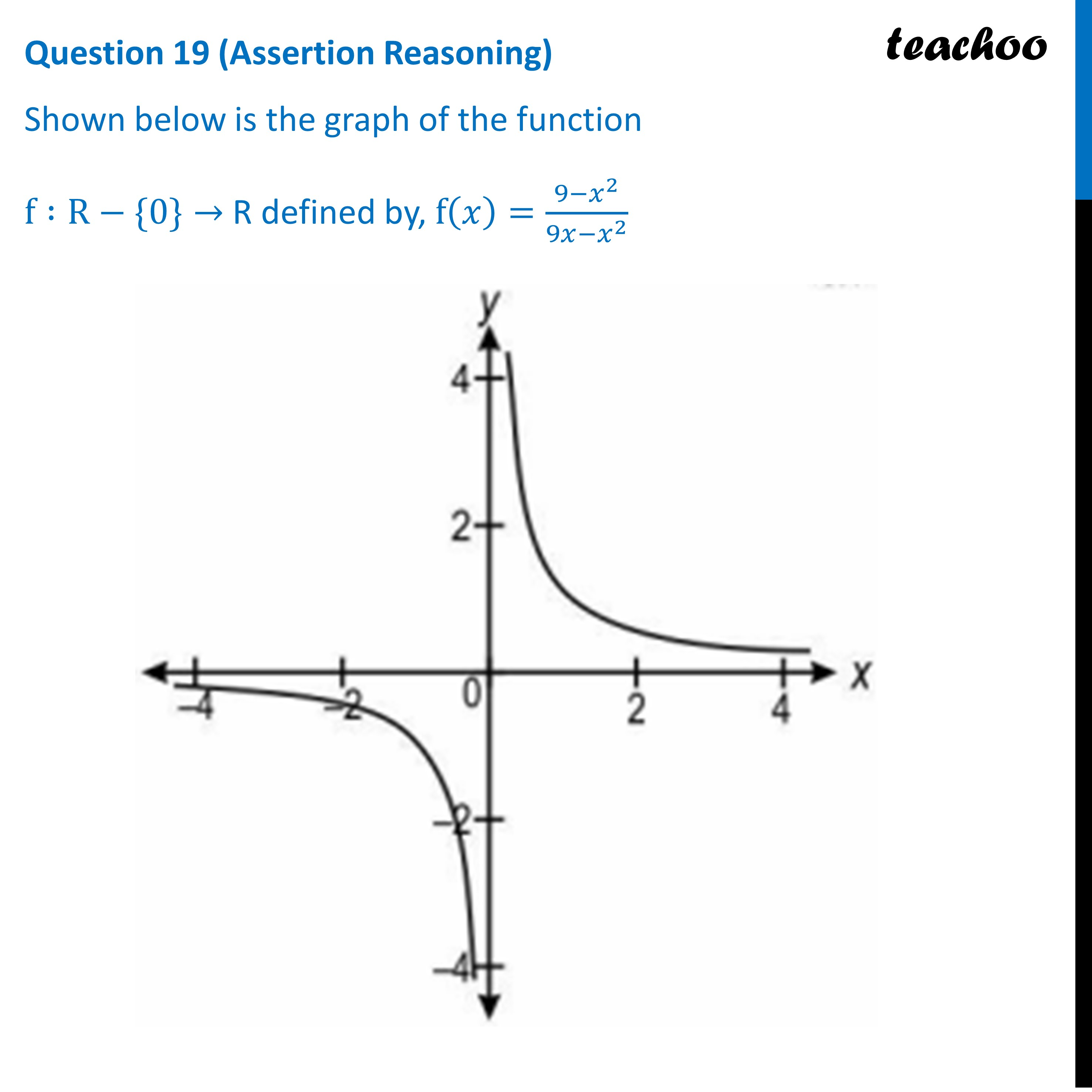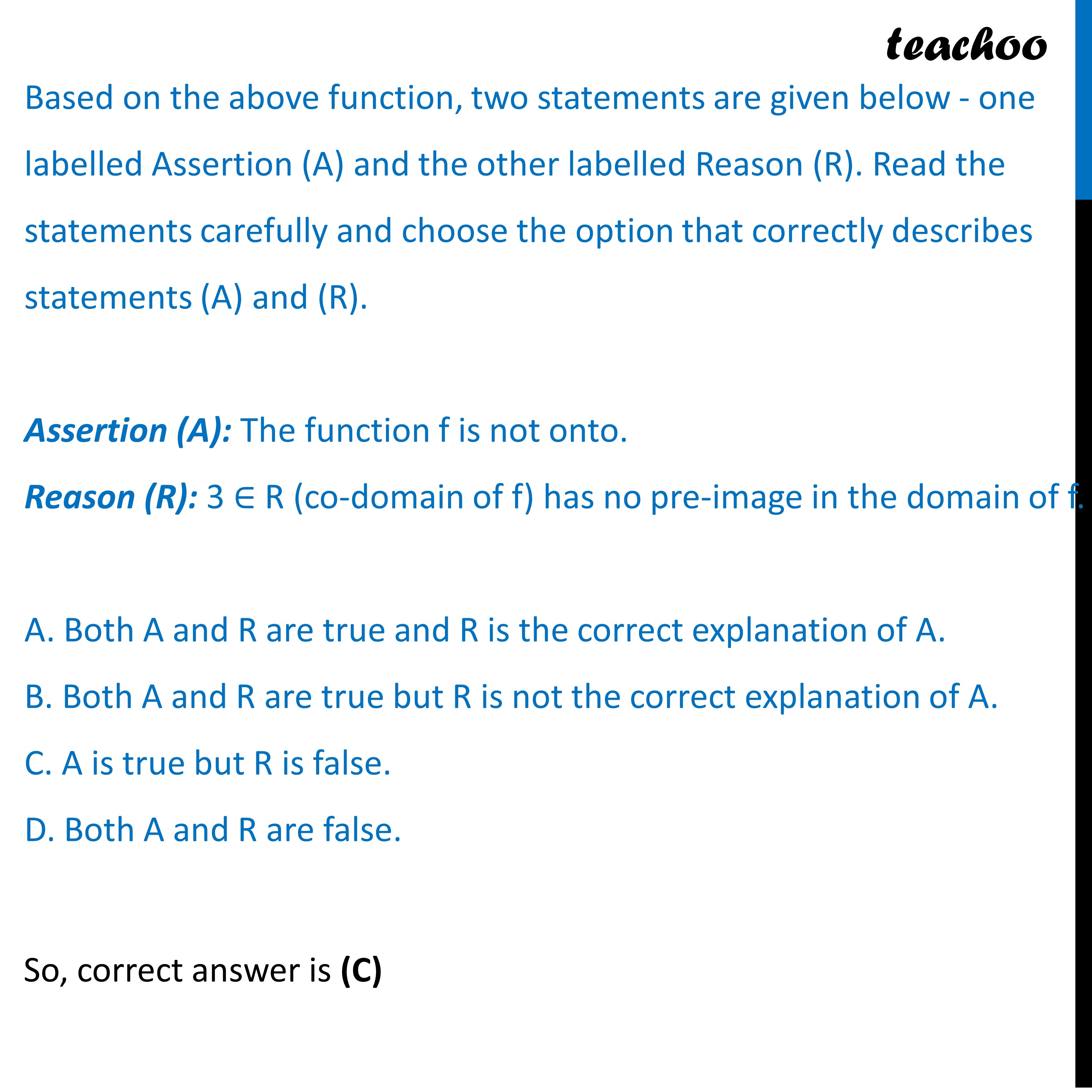Practice Questions CBSE - Maths Class 12 (2023 Boards)

Class 12
Solutions of Sample Papers and Past Year Papers - for Class 12 Boards

## D. Both A and R are false.Learn in your speed, with individual attention - Teachoo Maths 1-on-1 Class

### Transcript

Shown below is the graph of the function f :R−{0}→ R defined by, f(𝑥)=(9−𝑥^2)/(9𝑥−𝑥^2 ) Based on the above function, two statements are given below - one labelled Assertion (A) and the other labelled Reason (R). Read the statements carefully and choose the option that correctly describes statements (A) and (R). Assertion (A): The function f is not onto. Reason (R): 3 ∈ R (co-domain of f) has no pre-image in the domain of f. A. Both A and R are true and R is the correct explanation of A. B. Both A and R are true but R is not the correct explanation of A. C. A is true but R is false. D. Both A and R are false.